• ## C语言求定积分

万次阅读 2016-06-14 12:34:17
一个函数的定积分，其实就是它的面积，如函数 积分。 也就是要求出绿色部分的面积，如下： 我们可以通过矩形的方法来无限逼近定积分的求解，如下： 因为被分成n等分，就可以认为每一等分是一个...
题目要求：
求下面函数的定积分：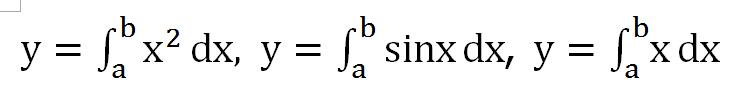思路：
求一个函数的定积分，其实就是求它的面积，如对函数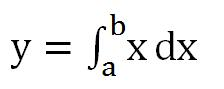求积分。
也就是要求出绿色部分的面积，如下：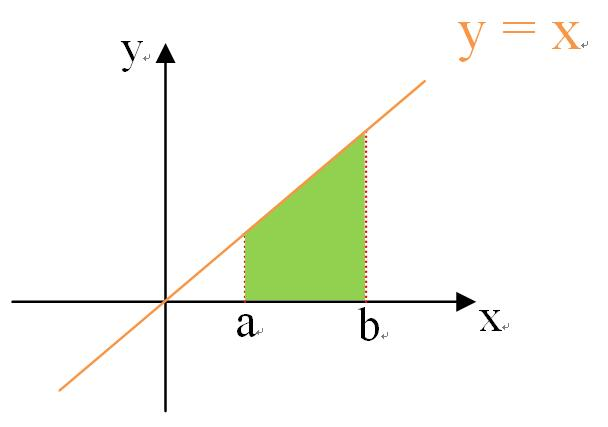我们可以通过矩形的方法来无限逼近定积分的求解，如下：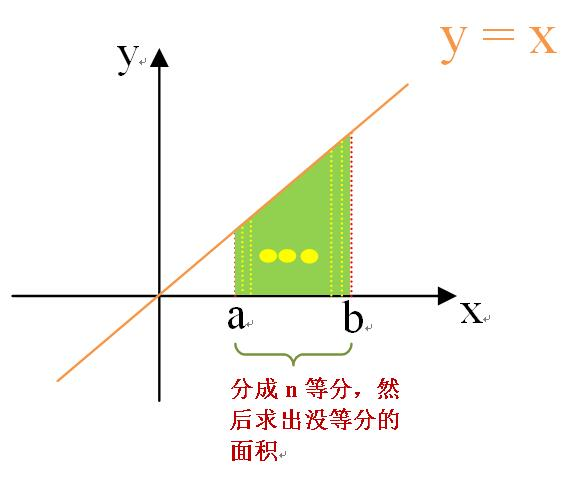因为被分成n等分，就可以认为每一等分是一个矩形，那么每一矩形的面积为： 每一个矩形面积为：***Sn=f(x)(b-a)/n 总面积为：****S=S1+S2+…+Sn
通过这样的一个思路就可以完成，定积分的求解。
这样这三个定积分的求解代码，如下：

#include <stdio.h>
#include <math.h>

void main( )
{
float f1(float x);
float f2(float x);
float f3(float x);
float s1 = 0;
float s2 = 0;
float s3 = 0;
int n;  //  被分成的份数
float a,b;  //   积分范围
int i;      // 循环变量

printf("请输入极限下限：");
scanf("%f",&a);
printf("请输入极限上限：");
scanf("%f",&b);
printf("请输入被分成的份数：");
scanf("%d",&n);
for(i=1; i<=n;i++)
{
s1 = s1 + f1(a+((b-a)/n)*i)*((b-a)/n);
s2 = s2 + f2(a+((b-a)/n)*i)*((b-a)/n);
s3 = s3 + f3(a+((b-a)/n)*i)*((b-a)/n);
}
printf("积分的结果为：%0.4f\n",s1);
printf("积分的结果为：%0.4f\n",s2);
printf("积分的结果为：%0.4f\n",s3);
}

/* y=x */
float f1(float x)
{
float y=0;
y = x;

return y;

}

/* y = x*x */
float f2(float x)
{
float y=0;
y = x*x;
return y;
}

/* y = sin(x) */
float f3(float x)
{
float y=0;
y = (float)sin(x);
return y;
}



结果为：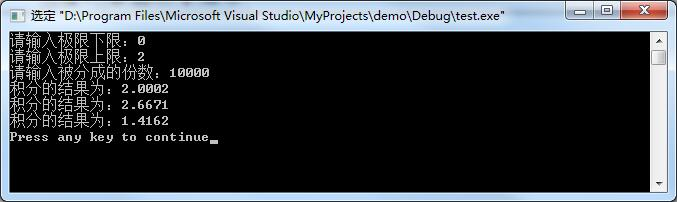这样就把定积分求完了，当然还有其他的方法。
展开全文• ## Python求积分（定积分）

万次阅读 多人点赞 2018-06-04 22:28:01
函数 ∫21xdx∫12xdx\int_1^2 {x} \,{d}x 代码 from sympy import * x = symbols('x') print(integrate(x, (x, 1, 2))) 解释 integrate(函数，（变量，下限， 上限）)
函数
$\int_1^2 {x} \,{d}x$
代码
from sympy import *
x = symbols('x')
print(integrate(x, (x, 1, 2)))

解释

integrate(函数，（变量，下限， 上限）)展开全文python
• ## 求定积分 c++实现

千次阅读 2018-12-02 15:59:05
本文用C++实现了一个简单函数的定积分 （目前仅支持一元四则混合运算）   说明： 求定积分部分采用的是辛普森积分法 表达式匹配部分采用的是表达式二叉树计算 code： /* Date:2018.12.2 Author:DeepWave ...
本文用C++实现了求一个简单函数的定积分

（目前仅支持一元四则混合运算）

说明：

求定积分部分采用的是辛普森积分法

表达式匹配部分采用的是表达式二叉树计算

code：

/*
Date:2018.12.2
Author:DeepWave
*/
#include<bits/stdc++.h>
using namespace std;
#define Var map<string,double>
const double eps = 1e-12;  //精度设置
Var vars;   //变量预定义
string str;  //表达式
string variable;  //变量名
double a, b;  //积分上下限

class Expression;
class Constant;
class UnkownNumber;
class Operation;
class Integral;
Expression *expressionTree(string s, int start, int len);
void Initialization();

class Expression
{
public:
virtual double Evaluate(Var v)
{
return 0;
}
};
Expression *EXP;  //表达式树

/*
常数类
*/
class Constant : public Expression
{
public:
double value;
Constant(double value)
{
this->value = value;
}
double Evaluate(Var vars)
{
return value;
}
};

/*
操作符类
由传入的左右子表达式计算
*/
class Operation: public Expression
{
public:
char op;
Expression *Left, *Right;
Operation(Expression *Left, Expression *Right, char op)
{
this->Left = Left;
this->Right = Right;
this->op = op;
}
double Evaluate(Var v)
{
double x = Left->Evaluate(v);
double y = Right->Evaluate(v);
switch(op)
{
case'+':
return x + y;
case'-':
return x - y;
case'*':
return x * y;
case'/':
return x / y;
}
return 0;
}
};

/*
未知数类
将表达式中的未知数进行转换
*/
class UnkownNumber: public Expression
{
public:
string name;
UnkownNumber(string name)
{
this->name = name;
}
double Evaluate(Var v)
{
return vars[name];
}
};

/*
由字符串转为表达式树
*/
Expression *expressionTree(string s, int start, int len)
{
bool hasAlpha = false, hasOperator = false;
int bracket = 0, PS = -1, MD = -1;

while(start <= len && s[start] == '(' && s[len] == ')')
start++, len--;
if(start > len)
return new Constant(0);

for(int i = start; i <= len; i++)
{
if(s[i] != '.' && !isdigit(s[i]))
{
if(isalpha(s[i]))
hasAlpha = true;
else
{
hasOperator = true;
switch(s[i])
{
case '+':
case '-':
if(!bracket) PS = i;
break;
case '*':
case '/':
if(!bracket) MD = i;
break;
case '(':
bracket++;
break;
case ')':
bracket--;
break;
}
}
}
}

if(hasAlpha == false && hasOperator == false)
return new Constant(stod(s.substr(start, len - start + 1)));
else if(hasOperator == false)
return new UnkownNumber(s.substr(start, len - start + 1));
else
{
int mid = PS == -1 ? MD : PS;
return new Operation(expressionTree(s, start, mid - 1), expressionTree(s, mid + 1, len), s[mid]);
}
return 0;
}

/*
积分类
*/
class Integral
{
public:
double a, b;
Integral(double a, double b)
{
this->a = a;
this->b = b;
}
double Function(double x)
{
vars[variable]=x;
return EXP->Evaluate(vars);
}
double simpson(double a, double b)
{
double c = a + (b - a) / 2;
return (Function(a) + 4 * Function(c) + Function(b)) * (b - a) / 6.0;
}
double calculate(double a, double b, double Eps)
{
double c = a + (b - a) / 2;
double mid = simpson(a, b);
double Left = simpson(a, c);
double Right = simpson(c, b);
if(fabs(Left + Right - mid) <= 15.0 * eps)
return Left + Right + (Left + Right - mid) / 15.0;
return calculate(a, c, Eps / 2.0) + calculate(c, b, Eps / 2.0);
}
double Evaluate(double Eps)
{
double up, down;
down = this->a;
up = this->b;
return calculate(down, up, Eps);
}
};

void Initialization()  //可预设常量值
{
vars["e"] = 2.7182818284;
vars["pi"] = acos(-1);
}

int main()
{
Initialization();
cout << "Please enter an expression that requires integration.(linear equation with one unknown)" << endl;
cin >> str;
EXP = expressionTree(str, 0, str.length() - 1);
cout << "Please enter the upper and lower limit of the integral." << endl;
cin >> a >> b;
cout << "Please input variable name." << endl;
cin >> variable;
Integral I (a, b);
printf("%.5f\n", I.Evaluate(eps));
return 0;
}


展开全文计算器 C
• ## 用MATLAB求定积分

万次阅读 多人点赞 2017-12-21 10:57:20
一、符号积分 符号积分由函数int来实现。该函数的一般调用格式为： int(s)：没有指定积分变量和积分阶数时，系统按findsym函数指示的默认变量被积函数或...该函数被积函数区间[a,b]上的定积分。a和b可以是两个


一、符号积分
符号积分由函数int来实现。该函数的一般调用格式为：
int(s)：没有指定积分变量和积分阶数时，系统按findsym函数指示的默认变量对被积函数或符号表达式s求不定积分；
int(s,v)：以v为自变量，对被积函数或符号表达式s求不定积分；
int(s,v,a,b)：求定积分运算。a,b分别表示定积分的下限和上限。该函数求被积函数在区间[a,b]上的定积分。a和b可以是两个具体的数，也可以是一个符号表达式，还可以是无穷(inf)。当函数f关于变量x在闭区间[a,b]上可积时，函数返回一个定积分结果。当a,b中有一个是inf时，函数返回一个广义积分。当a,b中有一个符号表达式时，函数返回一个符号函数。
例：
求函数x^2+y^2+z^2的三重积分。内积分上下限都是函数，对z积分下限是sqrt(x*y)，积分上限是x^2*y；对y积分下限是sqrt(x)，积分上限是x^2；对x的积分下限1，上限是2，求解如下：
>>syms x y z  %定义符号变量
>>F2=int(int(int(x^2+y^2+z^2,z,sqrt(x*y),x^2*y),y,sqrt(x),x^2),x,1,2)  %注意定积分的书写格式
F2 =
1610027357/6563700-6072064/348075*2^(1/2)+14912/4641*2^(1/4)+64/225*2^(3/4)    %给出有理数解>>VF2=vpa(F2)  %给出默认精度的数值解
VF2 =
224.92153573331143159790710032805
二、数值积分
1.数值积分基本原理
求解定积分的数值方法多种多样，如简单的梯形法、辛普生(Simpson)法、牛顿－柯特斯(Newton-Cotes)法等都是经常采用的方法。它们的基本思想都是将整个积分区间[a,b]分成n个子区间[xi,xi+1]，i=1,2,…,n，其中x1=a，xn+1=b。这样求定积分问题就分解为求和问题。
2.数值积分的实现方法
其中fname是被积函数名。a和b分别是定积分的下限和上限。tol用来控制积分精度，缺省时取tol=0.001。trace控制是否展现积分过程，若取非0则展现积分过程，取0则不展现，缺省时取trace=0。返回参数I即定积分值，n为被积函数的调用次数。
例：
求函数'exp(-x*x)的定积分，积分下限为0，积分上限为1。
>>fun=inline('exp(-x.*x)','x');  %用内联函数定义被积函数fname
Isim =
0.746824180726425
IL =
0.746824133988447
三、梯形法求向量积分
trapz(x,y)—梯形法沿列方向求函数Y关于自变量X的积分(向量形式，数值方法)。
>>d=0.001;
>>x=0:d:1;
>>S=d*trapz(exp(-x.^2))
S=
0.7468
或：
>>format long g
>>x=0:0.001:1;  %x向量，也可以是不等间距
>>y=exp(-x.^2);   %y向量，也可以不是由已知函数生成的向量
>>S=trapz(x,y);   %求向量积分
S =
0.746824071499185

int的积分可以是定积分，也可以是不定积分（即有没有积分上下限都可以积）可以得到解析的解，比如你对x^2积分，得到的结果是1/3*x^3，这是通过解析的方法来解的。如果int(x^2,x,1,2)得到的结果是7/3


展开全文• ## 利用JAVA求定积分

千次阅读 2017-12-27 11:42:18
需求：编译一个自动计算定积分的函数 方法：根据定义，曲线面积，分成n个区间，即n个矩形，由于每个区间差都是一样的， 可作为一个矩形的宽，矩形的长为每个区间的中点对应的函数，长和宽的乘积就是 其中一个小...
• #include&lt;iostream&gt; #include&lt;cmath&gt; using namespace std; double djf(double a,double b,int n); double fun(double x);...请输入积分下限"&lt;&lt;endl;  ...
• 核心思想 1、圆柱体的体积=底面积×高 2、微元法：旋转体的横截面进行无穷次切割，把每个很薄的横截面看成圆柱体，最后区间进行积分 例题 ...旋转体体积
•函数 高等数学 应用
• ## matlab求定积分

千次阅读 2019-12-22 10:47:29
f区间[a,b]上t的定积分。 符号解：例如f=t^2*exp(-t);a=0,b=1 >> clear >> syms t%定义符号变量 >> f=t^2*exp(-t); >> int(f,t,0,1) ans = 2 - 5*exp(-1) 数值解：例如例如f=t^2*...
• 用蒙特卡洛方法计算定积分 1.原理 计算定积分 利用蒙特卡洛计算方法，核心步骤是取随机的 g(X1),………,g(Xn...计算导函数[10,15]上的定积分； 3.测试语言 Python 4.测试代码 #Monte Carlo Meth...
• 1. 定积分是怎么定义的？ 按照现在的语言就是∫abf(x)dx=∑f(x)dx\int_a^bf(x)dx=\sum f(x)dx∫ab​f(x)dx=∑f(x)dx，所以定积分最初是被定义成面积的。...定积分可以面积，我们已经知道了，但是...
•高等数学 函数
• matlab求定积分与不定积分 创建于2018-03-21 22:42 求定积分与不定积分是一件比较繁琐的事，但是我们可以借助matlab，下面与大家分享解决方法 材料/工具 matlab 不定积分 函数“xe^x”的不定积分 ...
• ## c#求定积分

千次阅读 2018-10-27 16:57:12
步骤： 1 安装和使用NuGet插件 教程链接：https://blog.csdn.net/qq_36456952/article/details/55252913 2 安装 C# 科学计算库 Math.NET Numerics 教程链接: ... ...注意：安...c# NuGet
• ## 定积分

千次阅读 2018-09-23 17:57:51
定积分的概念与性质 定积分的定义 定积分概念与性质二 例题 比较积分大小
• ## matlab int 求定积分

万次阅读 2018-06-28 11:21:29
该函数被积函数区间[a,b]上的定积分。a和b可以是两个具体的数，也可以是一个符号表达式，还可以是无穷(inf)。当函数f关于变量x闭区间[a,b]上可积时，函数返回一个定积分结果。当a,b中有一个是inf时，函数返回...
• 为了得到定积分的值，需要将连续的对象分割为容易求解的子对象，然后利用迭代法表达式反复操作。求定积分的方法有两种：矩形法和梯形法。 下面主要以梯形法为例具体讲解： 函数y=f(x)的图像如图所示 从图中...
• f(x) = sin(x)/x的不定积分和负无穷到正无穷的定积分 sin(x)/x的不定积分是信号函数sig ，负无穷到正无穷的定积分为pi import math import numpy as np import matplotlib.pyplot as plt from sympy import * #...
• 题目要求是这样的：编写一个用矩形法求定积分的通用函数，定积分，上下限b,a由用户自行输入。 其中sin函数可以利用math.h。 这题的代码不难，关键是求解的数学思想而并非编程技巧，所谓的“矩形法”求定积分，...C语言 算法
•函数 高等数学 应用
• ## c语言实现求定积分

千次阅读 2015-02-08 18:21:32
#include #include double dj(double Min,double Max,double flags=0.00001)//最大值，最小值，精度 ... //这里的是sin的 } return j; } int main() { printf("%.6lf",dj(0,3.1415926)); }c语言 printf 数学
• python scipy 函数定积分 """ 定积分处理 函数 起始==>终止的面积 """ import numpy as np import matplotlib.pyplot as mp import matplotlib.patches as mc import scipy.integrate as si # 定义多项式...
•C程序设计
• 使用Python实现定积分、二重定积分（简单实用） 1.概述 最近项目需要使用程序实现数学微积分，最初想用java实现，后来发现可用文档太少，实现比较麻烦，后来尝试使用python实现，代码量较少，主要有sympy与scipy两种...python微积分
• 用蒙特卡洛方法求定积分   n=10; for i=1:4 %4次模拟 point=n.^i;%模拟的随机点数 RandData=rand(2,point); %产生x~[0,1],y~[0,1]上的随机数 scatter(RandData(1,:),RandData(2,:)) Below=find(Ran......